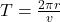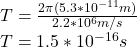## In the Bohr model of the hydrogen atom (see Section 38.5), in the lowest energy state the electron orbits the proton at a speed of 2.2 * 106

Question

In the Bohr model of the hydrogen atom (see Section 38.5), in the lowest energy state the electron orbits the proton at a speed of 2.2 * 106 m/s in a circular orbit of radius 5.3 * 10-11 m. (a) What is the orbital period of the electron?

in progress 0
1 week 2021-09-05T07:43:58+00:00 1 Answers 0 views 0

Orbital period of electron is 1.5×10⁻¹⁶s

Explanation:

Given data

Proton speed v=2.2×10⁶m/s

To find

Orbital period of electron T

Solution

We need to find the orbital period of electron the period is given by:Substitute the given valuesOrbital period of electron is 1.5×10⁻¹⁶s